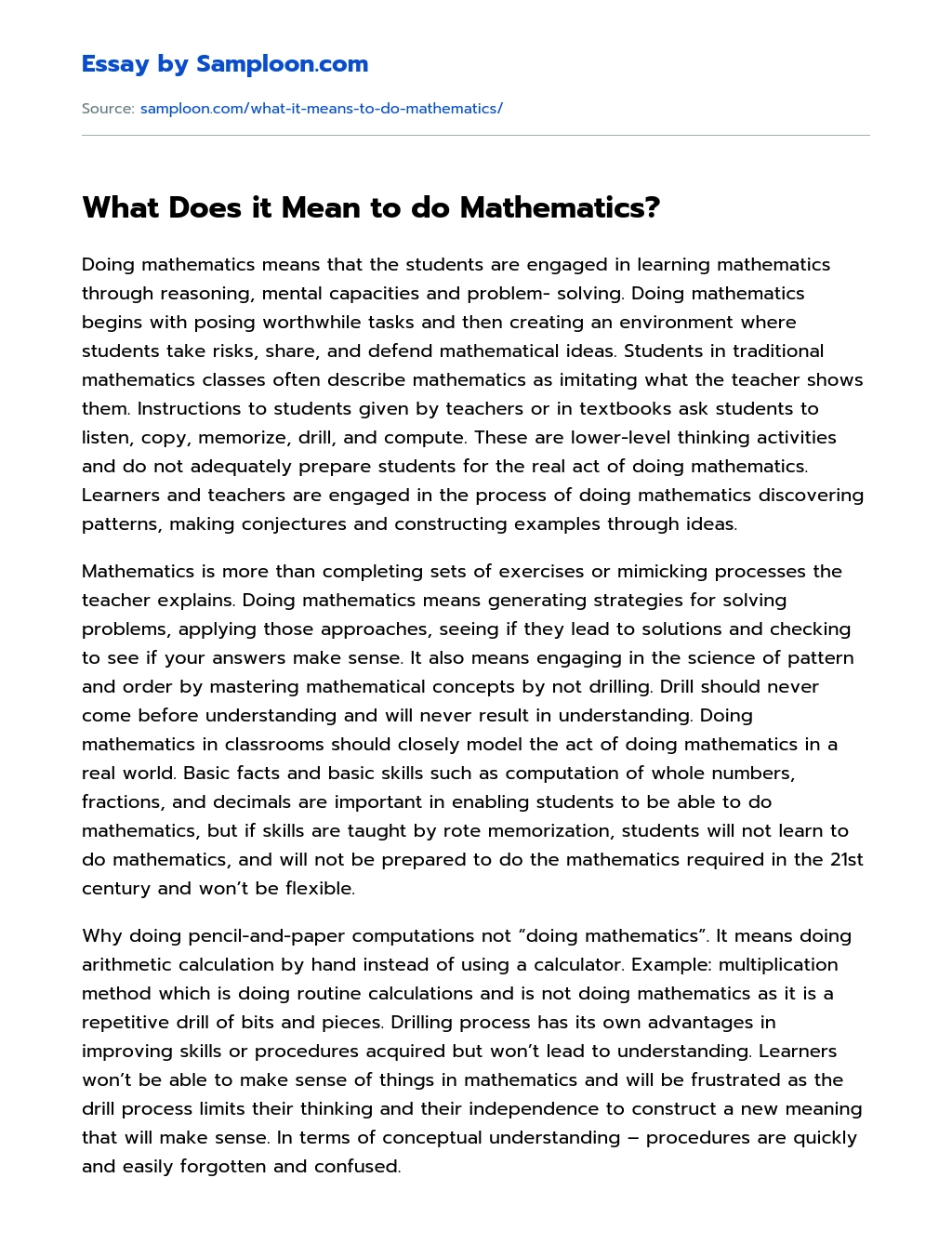# What Does it Mean to do Mathematics?

Updated October 13, 2020
• Subject
• Category
• Topic
• Pages 2
• Words 364
• Views 39Get help to write your own 100% unique essay

78 writers are online and ready to chat

Doing mathematics means that the students are engaged in learning mathematics through reasoning, mental capacities and problem- solving. Doing mathematics begins with posing worthwhile tasks and then creating an environment where students take risks, share, and defend mathematical ideas. Students in traditional mathematics classes often describe mathematics as imitating what the teacher shows them. Instructions to students given by teachers or in textbooks ask students to listen, copy, memorize, drill, and compute. These are lower-level thinking activities and do not adequately prepare students for the real act of doing mathematics. Learners and teachers are engaged in the process of doing mathematics discovering patterns, making conjectures and constructing examples through ideas.

Mathematics is more than completing sets of exercises or mimicking processes the teacher explains. Doing mathematics means generating strategies for solving problems, applying those approaches, seeing if they lead to solutions and checking to see if your answers make sense. It also means engaging in the science of pattern and order by mastering mathematical concepts by not drilling. Drill should never come before understanding and will never result in understanding. Doing mathematics in classrooms should closely model the act of doing mathematics in a real world. Basic facts and basic skills such as computation of whole numbers, fractions, and decimals are important in enabling students to be able to do mathematics, but if skills are taught by rote memorization, students will not learn to do mathematics, and will not be prepared to do the mathematics required in the 21st century and won’t be flexible.

Why doing pencil-and-paper computations not “doing mathematics”. It means doing arithmetic calculation by hand instead of using a calculator. Example: multiplication method which is doing routine calculations and is not doing mathematics as it is a repetitive drill of bits and pieces. Drilling process has its own advantages in improving skills or procedures acquired but won’t lead to understanding. Learners won’t be able to make sense of things in mathematics and will be frustrated as the drill process limits their thinking and their independence to construct a new meaning that will make sense. In terms of conceptual understanding – procedures are quickly and easily forgotten and confused.Remember. This is just a sample

You can get your custom paper from our expert writers

What Does it Mean to do Mathematics?. (2020, Sep 07). Retrieved from https://samploon.com/what-it-means-to-do-mathematics/

xHi!
I'm Peter!

Would you like to get a custom essay? How about receiving a customized one?

Check it out